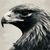# Getting unexpected georeferencing after raster processing in numpy?

174
2
03-14-2023 12:03 PMOccasional Contributor III

Hi, I'm attempting to run a simple canopy height model (CHM) using a surface and bare-earth terrain model.  I figured it would be quicker to do this in numpy, so I am using the RasterToNumPyArray methods in arcpy:

``````import arcpy
import os

arcpy.CheckOutExtensions('Spatial')
arcpy.env.overwriteOuptut = True

arcpy.env.outputCoordinateSystem = dsm
arcpy.env.cellSize = dsm

dsm_arr = arcpy.RasterToNumPyArray(dsm, nodata_to_value=0)
dtm_arr = arcpy.RasterToNumPyArray(dtm, nodata_to_value=0)

diff_arr = dsm_arr - dtm_arr
# Set things below 2m difference to 0
diff_arr[diff_arr < 2] = 0

diff = arcpy.NumPyArrayToRaster(diff_arr, value_to_nodata=0)

arcpy.CheckInExtension('Spatial')``````

The result is that the diff.tif output is way off.  The cell sizes are right, the SRS remains the same, and relatively speaking the values are correct (it's dropping negligible values I don't care about), but the georeferencing is off by thousands of miles.  What am I doing wrong?  Do I need to explicitly feed in a corner or extent?  If so, how would I do that?

Tags (2)
1 Solution

Accepted SolutionsbyMVP Esteemed Contributor

specify the lower left

NumPyArrayToRaster—ArcGIS Pro | Documentation

``````pnt = arcpy.Point(LL_X, LL_Y)
cell_size = # your cell size
no_data = # your nodata value or None
rast = arcpy.NumPyArrayToRaster(ai, pnt, cell_size, cell_size, no_data)``````

... sort of retired...
2 RepliesbyMVP Esteemed Contributor

specify the lower left

NumPyArrayToRaster—ArcGIS Pro | Documentation

``````pnt = arcpy.Point(LL_X, LL_Y)
cell_size = # your cell size
no_data = # your nodata value or None
rast = arcpy.NumPyArrayToRaster(ai, pnt, cell_size, cell_size, no_data)``````

... sort of retired...Occasional Contributor III

Thanks Dan, that did the trick.